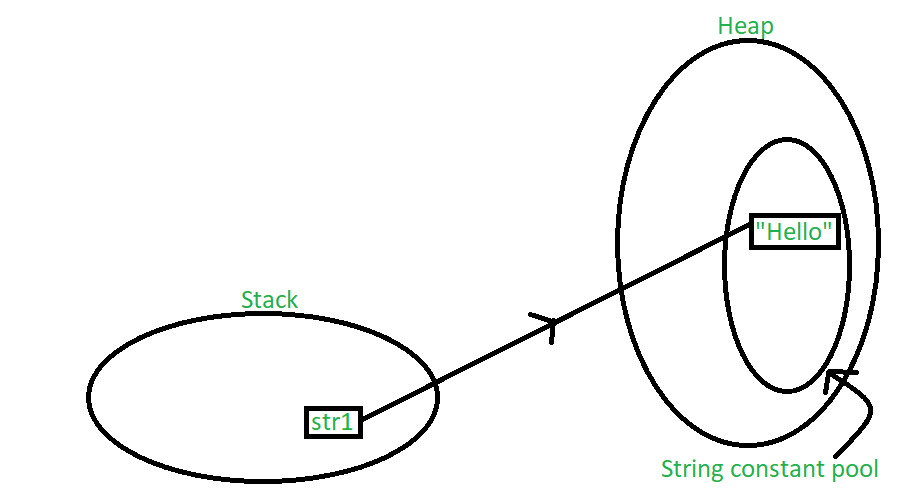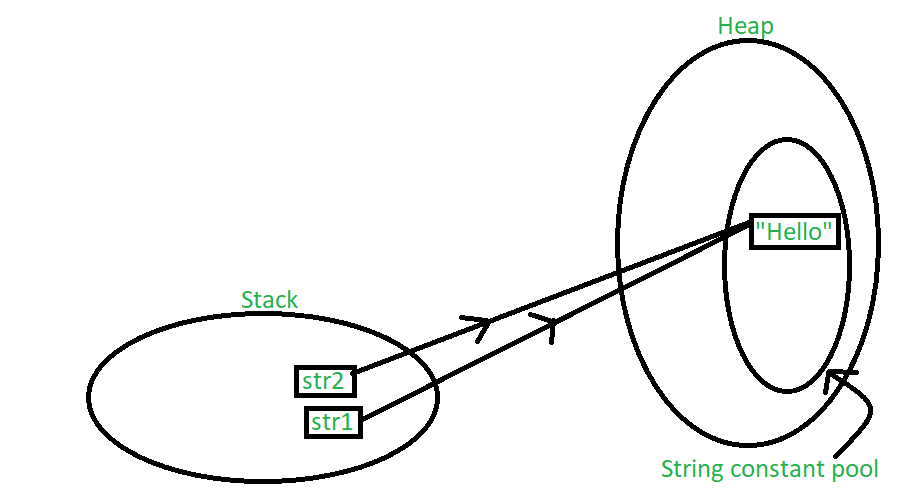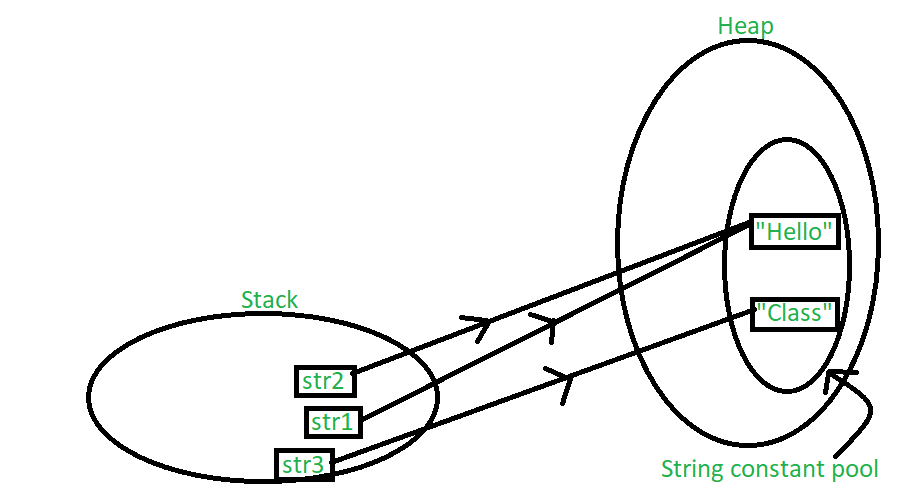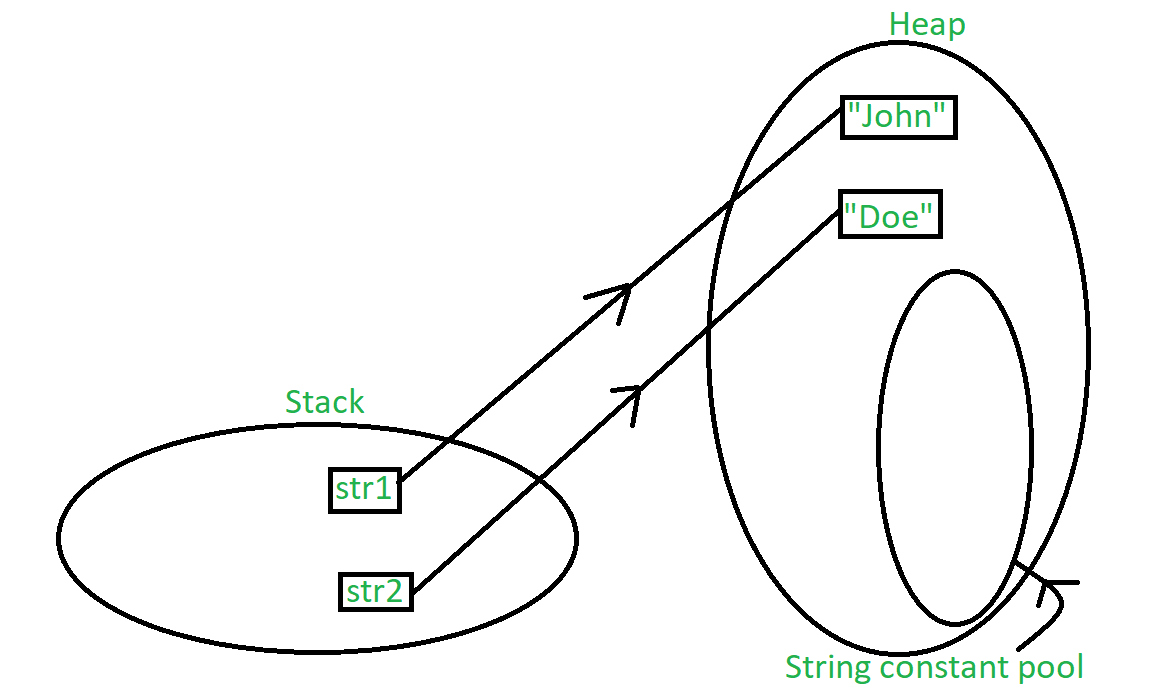Related Articles
String Constant Pool in Java
• Difficulty Level : Basic
• Last Updated : 21 Jun, 2020

Consider the following two Java programs, the first program produces output as “Yes”, but the second program produces output “No”. Can you guess the reason?

 `// Program 1: Comparing two references to objects ` `// created using literals. ` `import` `java.util.*; ` ` `  `class` `GFG { ` `    ``public` `static` `void` `main(String[] args) ` `    ``{ ` `        ``String s1 = ``"abc"``; ` `        ``String s2 = ``"abc"``; ` ` `  `        ``// Note that this == compares whether ` `        ``// s1 and s2 refer to same object or not ` `        ``if` `(s1 == s2) ` `           ``System.out.println(``"Yes"``); ` `        ``else` `           ``System.out.println(``"No"``); ` `    ``} ` `} `

Output:

```Yes
```

 `// Program 2: Comparing two references to objects ` `// created using new operator. ` `import` `java.util.*; ` ` `  `class` `GFG { ` `    ``public` `static` `void` `main(String[] args) ` `    ``{ ` `        ``String s1 = ``new` `String(``"abc"``); ` `        ``String s2 = ``new` `String(``"abc"``); ` ` `  `        ``// Note that this == compares whether ` `        ``// s1 and s2 refer to same object or not ` `        ``if` `(s1 == s2) ` `           ``System.out.println(``"Yes"``); ` `        ``else` `           ``System.out.println(``"No"``); ` `    ``} ` `} `

Output:

```No
```

Let us understand why we get different output with below explanation.

String is a sequence of characters. One of the most important characteristics of a string in Java is that they are immutable. In other words, once created, the internal state of a string remains the same throughout the execution of the program. This immutability is achieved through the use of a special string constant pool in the heap. In this article, we will understand about the storage of the strings.

A string constant pool is a separate place in the heap memory where the values of all the strings which are defined in the program are stored. When we declare a string, an object of type String is created in the stack, while an instance with the value of the string is created in the heap. On standard assignment of a value to a string variable, the variable is allocated stack, while the value is stored in the heap in the string constant pool. For example, let’s assign some value to a string str1. In java, a string is defined and the value is assigned as:

```String str1 = "Hello";
```

The following illustration explains the memory allocation for the above declaration:In the above scenario, a string object is created in the stack, and the value “Hello” is created and stored in the heap. Since we have normally assigned the value, it is stored in the constant pool area of the heap. A pointer points towards the value stored in the heap from the object in the stack. Now, let’s take the same example with multiple string variables having the same value as follows:

```String str1 = "Hello";
String str2 = "Hello";
```

The following illustration explains the memory allocation for the above declaration:In this case, both the string objects get created in the stack, but another instance of the value “Hello” is not created in the heap. Instead, the previous instance of “Hello” is re-used. The string constant pool is a small cache that resides within the heap. Java stores all the values inside the string constant pool on direct allocation. This way, if a similar value needs to be accessed again, a new string object created in the stack can reference it directly with the help of a pointer. In other words, the string constant pool exists mainly to reduce memory usage and improve the re-use of existing instances in memory. When a string object is assigned a different value, the new value will be registered in the string constant pool as a separate instance. Lets understand this with the following example:

```String str1 = "Hello";
String str2 = "Hello";
String str3 = "Class";
```

The following illustration explains the memory allocation for the above declaration:One way to skip this memory allocation is to use the new keyword while creating a new string object. The ‘new’ keyword forces a new instance to always be created regardless of whether the same value was used previously or not. Using ‘new’ forces the instance to be created in the heap outside the string constant pool which is clear, since caching and re-using of instances isn’t allowed here. Let’s understand this with an example:

```String str1 = new String("John");
String str2 = new String("Doe");
```

The following illustration explains the memory allocation for the above declaration:Attention reader! Don’t stop learning now. Get hold of all the important Java Foundation and Collections concepts with the Fundamentals of Java and Java Collections Course at a student-friendly price and become industry ready.

My Personal Notes arrow_drop_up
Recommended Articles
Page :